# Boolean Algebra and Logic Simplification

## Boolean Algebra and Logic SimplificationLOGIC SIMPLIFICATION

1) LAWS AND RULES OF BOOLEAN ALGEBRA

=> Laws of Boolean Algebra

## Commutative Laws

1) The commutative law of addition for two variables is written as

A+B = B+A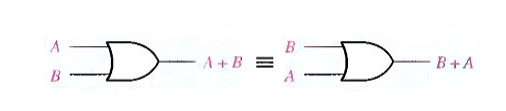Fig 1: Application of commutative law of addition.

2)The commutative law of multiplication for two variables is A.B = B.A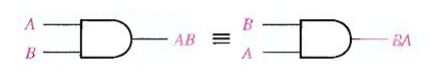Fig.2 Application of commutative law of multiplication

# Fig.5 Application of distributive law.

Basic rules of Boolean algebra.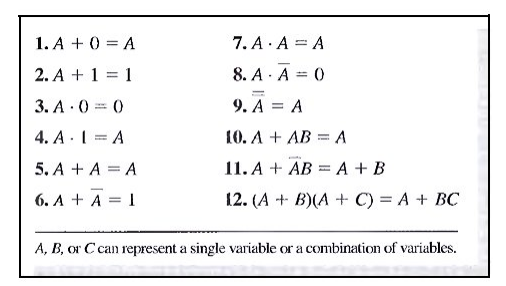Rule 1. A + 0 = A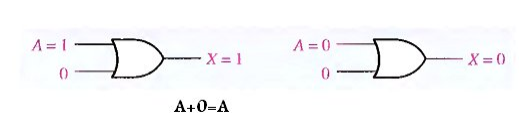Rule 2. A + 1 = 1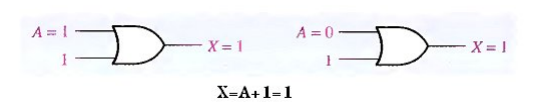Rule 3. A . 0 = 0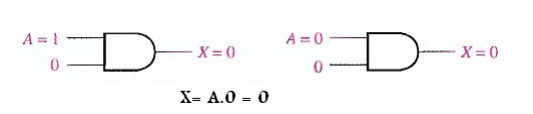Rule 4. A . 1 = A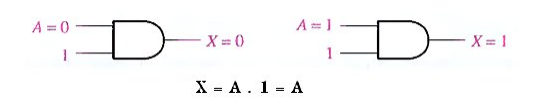Rule 5. A + A = A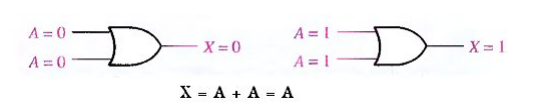Rule 6. A + A = 1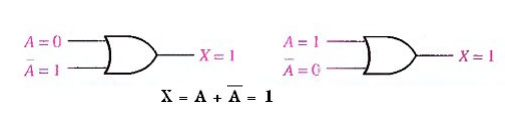Rule 7. A . A = A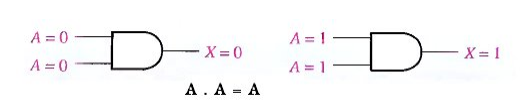Rule 8. A . A = 0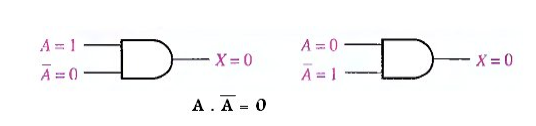Rule 9 A = A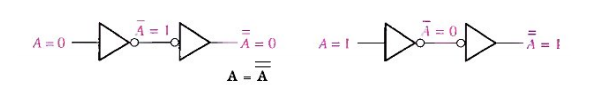Rule 10. A + AB = A

This rule can be proved by applying the distributive law,

A + AB = A( 1 + B)                        distributive law

= A . l                                             (1 + B) = 1

= A                                                 A . 1 = A

The proof is shown in Table below  which shows the truth table and the resulting logic circuit simplification.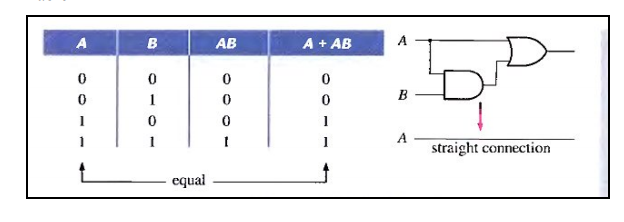Rule 11. A + AB = A + B

Take LHS

A + AB = (A + AB) + AB              since A = A + AB

= (AA + AB) + AB            since A = AA

=AA +AB +AA +AB         adding AA = 0

= (A + A) (A + B)              Factoring = 1. (A + B)

=A + B                               A + A = 1

The proof is shown in Table 4-3, which shows the truth table and the resulting logic circuit simplification.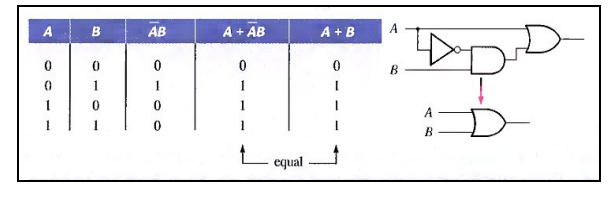Rule 12. (A + B)(A + C) = A + BC

Take LHS

(A + B)(A + C) = AA + AC + AB + BC                  Distributive law

= A( 1 + C) + AB + BC

= A. 1 + AB + BC                            1 + C = 1 Factoring (distributive law)

= A(1 + B) + BC                              = A. 1 + BC

= A + BC                                          A . 1 = A   and     1 + B = 1

The proof is shown in Table below , which shows the truth table and the resulting logic circuit simplification.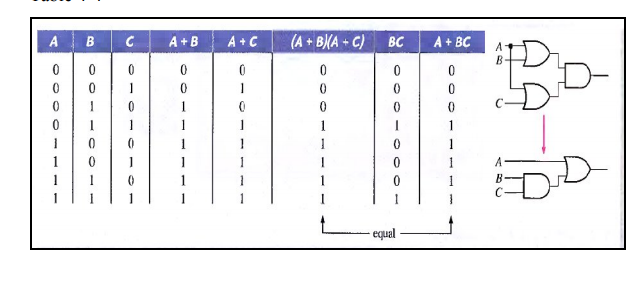• Simplification Of Boolean Expression Using Boolean Algebra

1. A simplified Boolean expression uses the fewest gates possible to implement a given expression.

Using Boolean algebra techniques, simplify this expression: AB + A(B + C) + B(B + C)

Solution